# Learning OpenCV 3 Application Development4.7 (3 reviews total)
By Samyak Datta
• \$41.99 eBook
• \$51.99 Print + eBook
• \$12.99 eBook + Subscription
What do you get with a Packt Subscription?

• Constantly updated with 100+ new titles each month
• Breadth and depth in over 1,000+ technologies

Computer vision and machine learning concepts are frequently used in practical computer vision based projects. If you’re a novice, this book provides the steps to build and deploy an end-to-end application in the domain of computer vision using OpenCV/C++.

At the outset, we explain how to install OpenCV and demonstrate how to run some simple programs. You will start with images (the building blocks of image processing applications), and see how they are stored and processed by OpenCV. You’ll get comfortable with OpenCV-specific jargon (Mat Point, Scalar, and more), and get to know how to traverse images and perform basic pixel-wise operations.

Building upon this, we introduce slightly more advanced image processing concepts such as filtering, thresholding, and edge detection. In the latter parts, the book touches upon more complex and ubiquitous concepts such as face detection (using Haar cascade classifiers), interest point detection algorithms, and feature descriptors. You will now begin to appreciate the true power of the library in how it reduces mathematically non-trivial algorithms to a single line of code!

The concluding sections touch upon OpenCV’s Machine Learning module. You will witness not only how OpenCV helps you pre-process and extract features from images that are relevant to the problems you are trying to solve, but also how to use Machine Learning algorithms that work on these features to make intelligent predictions from visual data!

Publication date:
December 2016
Publisher
Packt
Pages
310
ISBN
9781784391454

## Chapter 1. Laying the Foundation

Computer vision is a field of study that associates itself with processing, analyzing, and understanding images. It essentially tries to mimic what the human brain does with images captured by our retina. These tasks are easy for human beings but are not so trivial for a computer. In fact, some of them are computationally so challenging that they are open research problems in the computer vision community. This book is designed to help you learn how to develop applications in OpenCV/C++. So, what is an ideal place to start learning about computer vision? Well, images form an integral component in any vision-based application. Images are everywhere! Everything that we do in the realm of computer vision boils down to performing operations on images.

This chapter will teach you the basics of images. We will discuss some common jargons that come up frequently while we talk about images in the context of computer vision. You will learn about pixels that make up images, the difference between color spaces and color channels and between grayscale and color images. Knowing about images is fine, but how do we transfer our knowledge about images to the domain of a programming language? That's when we introduce OpenCV.

OpenCV is an open source computer vision and machine learning library. It provides software programmers an infrastructure to develop computer vision-based applications. The library has its own mechanisms for efficient storage, processing, and retrieval of these images. This will be a major topic of discussion in this chapter. We will learn about the different data structures that the OpenCV developers have made available to the users. We will try to get a glimpse of the possible use cases for each of the data structures that we discuss. Although we try to cover as many data structures as possible, for starters, this chapter will focus on one particular data structure of paramount importance, which lies at the core of everything that we can possibly do with the library: the Mat object.

At the most basic level, the Mat object is what actually stores the two-dimensional grid of pixel intensity values that represent an image in the digital realm. The aim of this chapter is to equip the readers with sufficient knowledge regarding the inner workings of the Mat object so that they are able to write their first OpenCV program that does some processing on images by manipulating the underlying Mat objects in the code. This chapter will teach you different ways to traverse your images by going over the pixels one by one and applying some basic modifications to the pixel values. The end result will be some cool and fascinating effects on your images that will remind you of filters in Instagram or any popular image manipulation app

We will be embarking on our journey to learn something new. The first steps are always exciting! Most of this chapter will be devoted to developing and strengthening your basics-the very same basics that will, in the near future, allow us to take up challenging tasks in computer vision, such as face detection, facial analysis, and facial gender recognition.

In this chapter, we will cover the following topics:

• Some basic concepts from the realm of digital images: pixels, pixel intensities, color depth, color spaces, and channels

• A basic introduction to the `Mat` class in OpenCV and how the preceding concepts are implemented in code using Mat objects

• A simple traversal of the `Mat` class objects, which will allow us to access as well as process the pixel intensity values of the image, one by one

• Finally, as a practical application of what we will learn during the chapter, we also present the implementations of some image enhancement techniques that use the pixel traversal concepts

## Digital image basics

Digital images are composed of a two-dimensional grid of pixels. These pixels can be thought of as the most fundamental and basic building blocks of images. When you view an image, either in its printed form on paper or in its digital format on computer screens, televisions, and mobile phones, what you see is a dense cluster of pixels arranged in a two-dimensional grid of rows and columns. Our eyes are of course not able to differentiate one individual pixel from its neighbor, and hence, images appear continuous to us. But, in reality, every image is composed of thousands and sometimes millions of discrete pixels.

Every single one of these pixels carries some information, and the sum total of all this information makes up the entire image and helps us see the bigger picture. Some of the pixels are light, some are dark. Each of them is colored with a different hue. There are grayscale images, which are commonly known as black and white images. We will avoid the use of the latter phrase because in image processing jargon, black and white refers to something else all together. It does not take an expert to deduce that colored images hold a lot more visual detail than their grayscale counterparts.

So, what pieces of information do these individual, tiny pixels store that enable them to create the images that they are a part of? How does a grayscale image differ from a colored one? Where do the colors come from? How many of them are there? Let's answer all these questions one by one.

### Pixel intensities

There are countless sophisticated instruments that aid us in the process of acquiring images from nature. At the most basic level, they work by capturing light rays as they enter through the aperture of the instrument's lens and fall on a photographic plate. Depending on the orientation, illumination, and other parameters of the photo-capturing device, the amount of light that falls on each spatial coordinate of the film differs. This variation in the intensity of light falling on the film is encoded as pixel values when the image is stored in a digital format. Therefore, the information stored by a pixel is nothing more than a quantitative measure of the intensity of light that illuminated that particular spatial coordinate while the image was being captured. What this essentially means is that any image that you see, when represented digitally, is reduced to a two-dimensional grid of values where each pixel in the image is assigned a numerical value that is directly proportional to the intensity of light falling on that pixel in the natural image.

### Color depth and color spaces

Now we come to the issue of encoding light intensity in pixel values. If you have studied a programming language before, you might be aware that the range and the type of values that you can store in any data structure are closely linked to the data type. A single bit can represent two values: 0 and 1. Eight bits (also known as a byte) can accommodate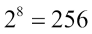different values. Going further along, an `int` (represented using 32 bits in most architectures) data type has the capacity to represent roughly 4.29 billion different entries. Extending the same logic to digital images, the range of values that can be used to represent the pixel intensities depends on the data type we select for storing the image. In the world of image processing, the term color space or color depth is used in place of data type.

The most common and simplest color space for representing images is using 8 bits to represent the value of each pixel. This means that each pixel can have any value between 0 and 255 (inclusive). Images made up of such color spaces are called grayscale images. By convention, 0 represents black, 255 represents white, and each of the other values between 0 and 255 stand for a different shade of gray. The following figure demonstrates such an 8-bit color space. As we move from left to right in the following figure, the grayscale values in the image gradually change from 0 to 255: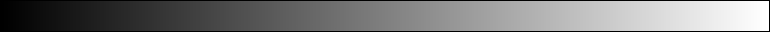So, if we have a grayscale image, such as the following one, then to a digital medium, it is merely a matrix of values-where each element of the matrix is a grayscale value between 0 (black) to 255 (white). This grid of pixel intensity values is represented for a tiny sub-section of the image (a portion of one of the wing mirrors of the car).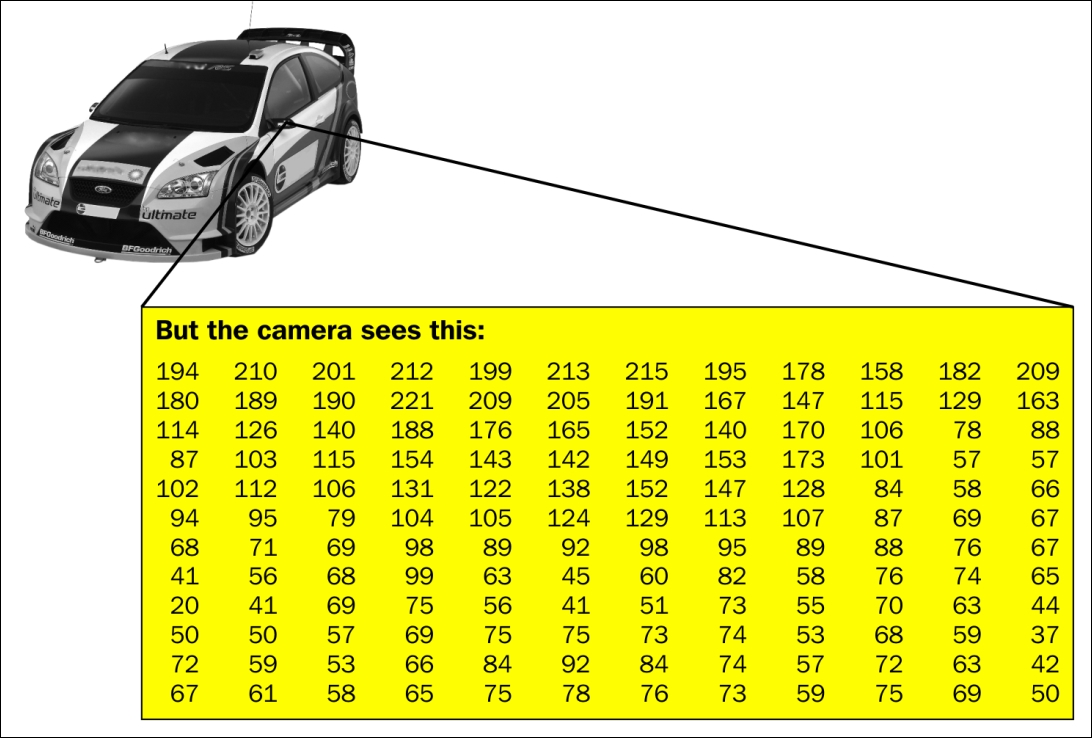### Color channels

We have seen that using 8 bits is sufficient to represent grayscale images in digital media. But how do we represent colors? This brings us to the concept of color channels. A majority of the images that you come across are colored as opposed to grayscale. In the case of the image we just saw, each pixel is associated with a single intensity value (between 0 and 255). For color images, each pixel has three values or components: the red (R), green (G), and blue (B) components. It is a well-known fact that all possible colors can be represented as a combination of the R, G, and B components, and hence, the triplet of intensity values at each pixel are sufficient to represent the entire spectrum of colors in the image. Also, note that each of the three R, G, and B values at every pixel are stored using 8 bits, which makes it 8 x 3 = 24 bits per pixel. This means that the color space now increases to more than 16 million colors from a mere 256. This is the reason color images store much more information than their grayscale counterparts.

Conceptually, the color image is not treated as having a triplet of intensity values at each pixel. Rather, a more convenient form of representation is adopted. The image is said to possess three independent color channels: the R, G, and B channels. Now, since we are using 8 bits per pixel per channel, each of the three channels are grayscale images in themselves!

## Introduction to the Mat class

We have discussed the formation and representation of digital images and the concept of color spaces and color channels at length. Having laid a firm foundation on the basic principles of image processing, we now turn to the OpenCV library and take a look at what it has got to offer us in terms of storing digital images! Just like pixels are the building blocks of digital images, the `Mat` class is the cornerstone of OpenCV programming. Any instantiation of the `Mat` class is called the Mat object.

Before we embark on a description of the Mat object, I would urge you to think, keeping in mind whatever we have discussed regarding the structure and representation of digital images, about how you would go about designing a data structure in C++ that could store images for efficient processing. One obvious solution that comes to mind is using a two-dimensional array or a vector of vectors. What about the data types? An `unsigned char` should be sufficient since we would rarely need to store values beyond the range of 0 to 255. How would you go about implementing channels? Perhaps we could have an array of two-dimensional grids to represent color images (getting a little complicated now, isn't it?).

The Mat object is capable of doing all of the preceding things that were described (and much more) in the most efficient manner possible! It lets you handle multiple color channels and different color spaces without you (the programmer) having to worry about the internal implementation details. Since the library is written in C++, it also lifts the burden of memory management from the hands of the user. So, all you've got to worry about is building your cool application and you can trust Mat (and OpenCV) to take care of the rest!

According to OpenCV's official documentation:

"The class Mat represents an n-dimensional dense numerical single-channel or multi-channel array."

We have already witnessed that digital images are two-dimensional arrays of pixels, where each pixel is associated with a numerical value from a predefined color space. This makes the Mat object a very obvious choice for representing images inside the world of OpenCV. And indeed, it does enable you to load, process, and store images and image data in your program. Most of the computer vision applications that you will be developing (as part of this book or otherwise) would involve abundant usage of images. These images would typically enter your system from the outside (user input), your application would apply several image processing algorithms on them, and finally produce an output, which may be written to disk. All these operations involve storing images inside your program and passing them around different modules of your code. This is precisely where the Mat object lends its utility.

Mat objects have two parts: a header and the actual matrix of pixel values. The header contains information, such as the size of the matrix, the memory address where it is stored (a pointer), and other pieces of information pertaining to the internal workings of Mat and OpenCV. The other part of the Mat object is where the actual pixel values are stored. The header for every Mat object is constant in size, but the size of the matrix of pixel values depends on the size of your image.

As this book progresses, you will realize that a Mat object is not always synonymous with images. You will work with certain instantiations of the `Mat` class, which do not represent a meaningful image as such. In such cases, it is more convenient to think of the Mat object as a data structure that helps us to operate on (possibly multidimensional) numerical arrays (as the official document suggests). But irrespective of whether we use Mat as an image store or a generic multidimensional array, you will soon realize the immense power that the creators of the library have placed in your hands through the `Mat` class. As mentioned earlier, its scope goes beyond merely storing images. It can act as a data structure and can provide the users with tools to use the most common linear algebra routines--matrix multiplication, inverse, eigen-values, PCA, norms, SVD, and even DFT and DCT--the list goes on.

We have covered enough theory without writing any actual code. And this is precisely what we are going to do now--explore OpenCV and try to learn more about the `Mat` class! We just read about the utility of Mat object both as a structure for storing images and as a multidimensional array. We'll start by witnessing the former. To that end, we will be writing our first Hello World OpenCV program that will read an image from the disk, load the image data onto a Mat object, and then display the image. All of this will be done using OpenCV and C++. So, let's begin!

At the very outset, we include the relevant header files and namespace declarations:

```#include <opencv2/highgui/highgui.hpp>
#include <opencv2/core/core.hpp>

using namespace std;
using namespace cv;
```

The `highgui` header contains declarations for the functions that do the following:

1. Read an image from disk and store it in a Mat object : `imread()`.

2. Display the contents of a Mat object (the image) onto a window : `imshow()`.

The `core.hpp` header file contains declarations for the `Mat` class. Now we come to the actual piece of code that performs the intended operations:

```int main() {
imshow("Output", image);
waitKey(0);

return 0;
}
```

The first thing we encounter in the code snippet is the `imread()` function. It basically allows you to read an image from the disk and load its contents on to a Mat object. It accepts a couple of arguments.

The first argument is the full path name of the image file on disk. Here, we pass `image.png` as our path (make sure to give the complete path here; if you just pass the name of the image as we have done, ensure that the file lies in the same directory as your code).

The second argument is an OpenCV flag that tells us the format in which to load the image onto the Mat object. The different possible flags along with their descriptions are given in the following table. Out of all these flags, you will be using a couple of them quite frequently: `IMREAD_UNCHANGED` and `IMREAD_GRAYSCALE`. The former loads the image as is, whereas the latter always converts the image into a single channel grayscale image.

 Flag Description `IMREAD_UNCHANGED` If set, return the loaded image as is `IMREAD_GRAYSCALE` If set, always convert the image to the single channel grayscale image `IMREAD_COLOR` If set, always convert the image to the three channel BGR color image `IMREAD_ANYDEPTH` If set, return the 16-bit/32-bit image when the input has the corresponding depth, otherwise convert it to 8-bit `IMREAD_ANYCOLOR` If set, the image is read in any possible color format `IMREAD_LOAD_GDAL` If set, use the `gdal` driver for loading the image

Then comes `imshow()`. It does the opposite of what `imread()` accomplishes. It takes the contents of a Mat object and displays it on the screen inside a window. This function also accepts two arguments:

1. The first argument is the name that appears in the title of the window that displays the image. Here, we have named the window `Output`.

2. The second argument is, of course, the Mat object which stores the image.

The `waitkey()` method pauses the program for a specified amount of time, waiting for a keystroke. If, however, we pass `0` as an argument, it would wait indefinitely for us to press the key. Had we not included the `waitKey(0)` statement in our code, the OpenCV window with the image would have flashed on our screens and disappeared, following which our program would have terminated `return 0`. Having the `waitKey(0)` after `imshow()` displays the image and then waits for the user to press a key, and only then does the program terminate.

## Exploring the Mat class - declaring Mat objects

We have just witnessed the creation of a Mat object by reading an image from disk. Is loading an existing image the only way to create Mat objects in code? Well, the answer is no. It would be prudent to assume that there are other ways to declare and initialize instances of the `Mat` class. In the subsequent sections, we will be discussing some of the methods in great detail. As we move along the discussions, we will touch upon the different aspects of digital images that we introduced at the beginning of this chapter. You will see how the concepts of spatial resolution (image dimensions), color spaces (bit depths or data types), and color channels are all elegantly handled by the `Mat` class.

Let's see a sample line of code that both declares and initializes a Mat object:

`Mat M(20, 15, CV_8UC3, Scalar(0,0,255));`

### Spatial dimensions of an image

The first two arguments define the dimensions of the data matrix, that is, rows and columns, respectively. So the previous example will create a Mat object with a data matrix comprising 20 rows and 15 columns, which means a total of 20 x 15 = 300 elements. Often, you will see `Mat` declarations where both of these values are combined into a single argument: the `Size` object. The `Size` object, more specifically, the `Size_` template class, is an OpenCV specific class that allows us to specify sizes for images and rectangles. It has two members: width and height. So, if you are using a `Size` object to specify the dimensions of a `Mat`, the height and width correspond to the number of rows and columns, respectively. The same `Mat` instantiation using a `Size` object is given as follows:

```Mat M(Size(15, 20), CV_8UC3, Scalar(0,0,255));
```

There are a couple of things that are noteworthy regarding the preceding line of code. First, note that the number of rows and columns are in the reverse order with respect to the previous instantiation. This is because the constructor for the `Size_` class accepts the arguments in this order-width and height. Second, note that although the class is templatized and named `Size_`, in the declaration, we simply use `Size`. This is due to the fact that OpenCV has defined some aliases as follows:

```typedef Size_<int> Size2i;
typedef Size2i Size;
```

This basically means that writing `Size` is equivalent to saying `Size2i`, which in turn is the same as `Size_<int>`.

### Color space or color depth

The next argument to the Mat declaration statement discussed earlier is for the type. This parameter defines the type of values that the data matrix of the Mat object would store. The choice of this parameter becomes important because it controls the amount of space needed to store the Mat object in memory. OpenCV has its own types defined. A mapping between the OpenCV types and C++ data types is given in the following table:

 Serial No. OpenCV type Equivalent C++ type Range 0 `CV_8U` `unsigned char` 0 to 255 1 `CV_8S` `char` -128 to 127 2 `CV_16U` `unsigned short` 0 to 65535 3 `CV_16S` `short` -32768 to 32767 4 `CV_32S` `int` -2147483648 to 2147483647 5 `CV_32F` `float` 6 `CV_64F` `double`

`16`, `32`, and `64` represent the number of bits used for storing a value of that data type. `U`, `S`, and `F` stand for `unsigned``short`, and `float`, respectively. Using these two pieces of information, we can easily deduce the range of values for each data type, as given in the right-most column of the table.

### Color channels

You will notice a `C` followed by a number in the types used to declare our Mat objects (for example, `CV_8UC3`). The `C` here stands for channel, and the integer following it gives you the number of channels in the image. Given a multi-channel, RGB image, OpenCV provides you with a `split()` function that separates the three channels. Here is a short code snippet that demonstrates this:

```Mat color_image = imread("lena.jpg", IMREAD_COLOR);
vector<Mat> channels;
split(color_image, channels);

imshow("Blue", channels);
imshow("Green", channels);
imshow("Red", channels);
waitKey(0);```

### Image size

By looking at the complete OpenCV type (along with the number of channels) and the Mat object dimensions, we can actually calculate the number of bits that would be required to store all the pixel values in memory. For example, let's say we have a 100 x 100 Mat object of type `CV_8UC3`. Each pixel value will take 8 bits and there will be three such values for each pixel (three channels). That takes it to 24 bits per pixel. There are 100 x 100 = 10,000 pixels in total, which means a total space of (24 x 10,000) bits = 30 kilobytes. Keep in mind that this is the space used up by the grid of pixel values and does not include the header. The overall size of the Mat object will be higher, but not by a significant amount (the size of the data matrix is substantially larger than the size of a `Mat` header).

By looking at the range of data types available for declaring Mat objects, it's natural to think about the utility of all the different types. For storing and representing images, only `CV_8UC1` and `CV_8UC3` make sense, the former for grayscale images and the latter for images in the RGB color space. As stated earlier, in OpenCV, the Mat object is used for much more than an image store. For applications where Mat is best treated as a multidimensional numerical array, the other types make sense. However, irrespective of whether the Mat object serves as an image store or as a data structure, its importance and ubiquity inside the world of OpenCV is undeniable.

### Default initialization value

The last argument is the default value for the data matrix of the Mat object. You will have noticed the use of yet another OpenCV specific data structure: `Scalar`. The `Scalar_` class allows you to store a vector of at most four values. You might be wondering about the utility of restricting the size of a vector to just four. There are several use cases within OpenCV where we might require working with one, two, or three values (and not more than that). For example, we have just learnt that each pixel in an RGB image is represented using three values, one each for the R, G, and B channels. In such a scenario, the `Scalar` object provides a convenient method to pass the group of three values to the Mat object constructor, as has been done in the example under consideration. One important thing to note is that OpenCV reads the color channels in the reverse order-B, G, and R. This means that passing `Scalar(255, 0, 0)` would refer to blue, whereas `Scalar(0, 0, 255)` is red. Any combination of the three would then represent one of the 16 million+ colors. If, at this point of time, you are wondering about providing default values for a grayscale image, OpenCV allows what is intuitive. A simple `Scalar(0)` or `Scalar(255)` will color all pixels black or white, respectively, by default in a grayscale image. What I mean to say is that the constructor for the `Scalar` object is flexible enough to accept one, two, three, or even four values. If you are wondering about the discrepancy in the class names, `Scalar_` and `Scalar`, similar to the `Size_` class, OpenCV defines the following alias to make our code less verbose:

```typedef Scalar_<double> Scalar;
```

The initialization method that we discussed here involved passing all the three pieces of information as arguments:

• Dimensions of the image

• The type of data stored at each pixel location

• The initial value to be filled in the data matrix

However, the `Mat` class allows greater flexibility in declaring objects. You do not have to specify all the three mentioned earlier. The `Mat` class has some overloaded constructors that allow you to declare objects even if you simply specify the following:

• Nothing at all

• The dimensions and the type

• The dimensions, the type, and the initial value

Here are some of the constructor declarations from the implementation of the `Mat` class:

```Mat ()
Mat (int rows, int cols, int type)
Mat (Size size, int type)
Mat (int rows, int cols, int type, const Scalar &s)
Mat (Size size, int type, const Scalar &s)
```

Going by the preceding definitions, we present some sample valid Mat object declarations. You will get a chance to see them being used in the programs that we write as part of this book:

```Mat I;
Mat I(100, 80, CV_8UC1);
Mat I(Size(80, 100), CV_8UC1);
```

Before we finish this section on declaring Mat objects, we will discuss one final technique, that is, creating Mat objects as a region of interest (ROI) from inside an existing Mat object. Often, situations arise where we are interested in a subset of the data from the data matrix of an existing Mat object. Putting it another way, we would like to initialize a new Mat object whose data matrix is a submatrix of the existing Mat object. The constructor for such an initialization is given as `Mat (const Mat &m, const Rect &roi)`. A sample statement that invokes such a constructor is given as follows:

```Mat roi_image(original_image, Rect(10, 10, 100, 100));
```

This will create a new Mat object named `roi_image` by taking the data from the matrix belonging to the existing Mat object, `original_image`. The submatrix will start from the pixel with coordinates `(10, 10)` as the upper-left corner and will have dimensions of 100 x 100. All the information pertaining to the size of the ROI has been passed via the `Rect` object, which is yet another OpenCV specific data structure.

## Digging inside Mat objects

We have learnt how to create Mat objects and even populate them with data from an image read from the disk or with arbitrary numerical values. Now it's time to get a little more information regarding the internal workings of the Mat object. This will help you make some important design decisions while writing code for your applications.

As we have discussed earlier, Mat objects are composed of a header and the actual matrix of values with the size of the matrix being (usually) much greater than the size of the header. We have already seen that a modestly sized image with dimensions of 100 pixels by 100 pixels can take up as much as 30 kilobytes of space. Images are known to be much bigger in size than that. Moreover, when you are developing a computer vision-based application, your code is typically working with multiple images or multiple copies of images. These images (and their copies) are passed to-and-fro the various modules of your code. They may be the input to or store the result of some OpenCV function. The more sophisticated a system we are trying to build, the greater the complexity of these interactions.

If that is the case, with Mat being a memory-intensive data structure, how does OpenCV prevent its processes from running out of memory? The answer to the question lies in the manner in which the internal workings of the Mat objects are handled by the library. OpenCV is smart enough to avoid duplication of the Mat object data matrix wherever it possibly can. This is going to be the topic of our discussions in this section.

We have discussed several ways to declare and initialize Mat objects. One more method we will touch upon now is by initializing it with another Mat object (much like what a copy constructor does). So, we can do something like this:

```Mat image = imread("lena.jpg");
Mat another_image(image);
Mat yet_another_image = image;
```

Now, your intuition might tell you that since there are three Mat objects, the data of the image read from the disk must have been duplicated three times in memory. Had that been the case, and had the original image, `lena.jpg`, contained a significant number of pixels, it would have meant using up a lot of memory. However, while using the copy constructor for Mat, OpenCV only creates a separate copy of the header and not the data matrix. Same is the case with using the equality operator. So, for all the three Mat objects, the header is different, but the data matrix is shared. The headers for each of the three objects point to the same data matrix in memory. In essence, we have three different aliases providing access to the same underlying data matrix. Modifying any of the three objects will change the same data and affect all three. It is very important to keep this in mind while writing code to avoid unnecessary complications and potential loss of data by overwriting!

Another place where such an issue might crop up is while passing images to functions in your code. Suppose you have a function in your application that looks something like this:

```void processImage(Mat image) {
// Does some processing on the Mat
}
```

When you invoke the preceding function, the `processImage()` method works on the same data matrix. Another way to put it is that Mat objects are always passed by reference (the actual data matrix is not copied). Therefore, modifying the image in the called function will modify it in the function from where it was called.

Let's test this using a concrete example that you can execute for your and check. We will start with the inclusion of the relevant header files and namespace declarations:

```#include <iostream>
#include <opencv2/core/core.hpp>
#include <opencv2/highgui/highgui.hpp>

using namespace std;
using namespace cv;
```

We have an implementation of the `processImage()` method that turns all the pixels of the input image black:

```void processImage(Mat input_image) {
int channels = input_image.channels();
int numRows = input_image.rows;
int numCols = input_image.cols * channels;

for (int i = 0; i < numRows; ++i) {
uchar* image_row = input_image.ptr<uchar>(i);
for (int j = 0; j < numCols; ++j)
image_row[j] = 0;
}
}
```

Don't worry if you aren't able to understand the meaning of these lines for now. Traversing Mat objects will be covered in the subsequent sections of this chapter. You can copy the code verbatim and it will execute just fine:

```int main() {
processImage(image);

imshow("Output", image);
waitKey(0);

return 0;
}
```

As you can see here in the `main()` function, we read an image from the disk (`lena.png`), loaded the image data into a Mat object named `image`, and then passed the same object to our `processImage()` method, which was defined previously. When we attempt to display the same Mat object using `imshow()`, we see that the image is now completely black (which is what the `processImage()` method was expected to do!). This means that the `processImage()` method has worked with the same data matrix as that of the input Mat object.

But what about the cases where you actually do want to copy the data matrix as well? OpenCV provides a couple of alternatives to achieve this. `copyTo()` and `clone`() are two methods belonging to the `Mat` class that allow you to create separate Mat objects by copying the data matrix along with the header. A typical use case for this might be when you want a copy of the original image to be preserved before sending the image through your processing pipeline:

```Mat cloned_image = image.clone();
Mat another_cloned_image;
image.copyTo(another_cloned_image);
```

Let's test this on our previous example. The `processImage()` method remains unchanged. We will modify the `main()` function to look like this:

```int main() {
Mat image_clone = image.clone();
processImage(image);

imshow("image", image);
imshow("image_clone", image_clone);
waitKey(0);

return 0;
}
```

Notice that now, we create a copy of the input Mat object's data matrix by invoking the `clone()` method. If you run this, the `image_clone` parameter is Mat object would have remained unchanged, whereas the original data matrix has undergone the modifications of the `processImage` method.

This finishes our discussion of the Mat object. We have been through all the topics that you might need to begin working with images in your code. In the next section, we take a dive in and start by iterating through these images and playing around with their pixel values. Having learnt about the image, we now move on to some processing.

## Traversing Mat objects

So far, you have learnt in detail about the `Mat` class, what it represents, how to initialize instances of the `Mat` class, and the different ways to create Mat objects. Along the way, we have also looked at some other OpenCV classes, such as `Size`, `Scalar`, and `Rect`. We have also successfully run our very first OpenCV Hello World program. Our sample program was fairly simplistic. It read an image from disk and loaded the contents into a Mat object. The real fun begins after this. In any application that you develop, you would typically be reading an image or images from a storage disk into your code and then apply image processing or computer vision algorithms to them. In this section, we will take our first steps towards starting with the processing aspect of things.

As we stated at the outset, an image is the sum total of its pixels. So, to understand any sort of processing that gets applied to images, we need to know how the pixel values would be modified as a result of the operations. This gives rise to the necessity of iterating over each and every pixel of a digital image. Now, since images are synonymous with Mat objects within the realm of OpenCV, we need a mechanism that allows us to iterate over all the values stored in the data matrix of a Mat. This section will discuss some techniques to do the same. We will present a couple of different ways to achieve such a traversal along with the pros and cons of using each approach. Once again, you will come to appreciate the utility of the `Mat` class when you encounter some more Mat member functions that have been made available to aid the programmer with this task.

### Continuity of the Mat data matrix

Before we start with the code for traversing Mat objects, we need to understand how (more precisely, in what order) the data matrix stores the pixel values in memory. To do that, we need to introduce the concept of continuity. A data matrix is said to be continuous if all its rows are stored at adjacent memory locations without any gap between the contents of two successive rows. If a matrix is not continuous, it is said to be non-continuous. Now, why do we care if our Mat object's underlying data matrix is continuous or not? Well, as it turns out that iterating a continuous data matrix is much faster than going over a non-continuous one because the former requires a smaller number of memory accesses. Having learnt about the benefits offered by the continuity property of a Mat object's data matrix, how do we take advantage of this feature in our applications? The answer to that can be found in the following code snippet:

```int channels = image.channels();
int num_rows = image.rows;
int num_cols = (image.cols * channels);

if (image.isContinuous()) {
num_cols = num_cols * num_rows;
num_rows = 1;
}
```

This piece of code achieves what I like to call flattening of the data matrix, and this is typically performed as a precursor to the actual image traversal. If the rows of the data matrix are indeed saved in contiguous memory locations, this means that we can treat the entire matrix as a single one-dimensional array. This array will have one row and the number of columns will be equal to `(numRows*numCols*numChannels)`, which is the total number of pixels in the image. The code snippet assumes that the image is an 8-bit Mat object. Also note that the flattening is performed only if the image is continuous.

In the case of non-continuous images, the value of `numRows` and `numCols` remain as they are read from the Mat object.

Matrices created by `imread()`, `clone()`, or a constructor will always be continuous. In fact, the only time a matrix will not be continuous is when it borrows data from an existing matrix. By borrowing data, I mean when a new matrix is created out of an ROI of a bigger matrix, for example:

```Mat big (200, 300, CV_8UC1);
Mat roi (big, Rect(10, 10, 100, 100));
Mat col = big.col(0);
```

Both matrices, `roi` and `col`, will be non-continuous as they borrow data from `big`.

### Image traversals

Now, we are ready for the actual traversal. As stated earlier, we will discuss a couple of different ways to go about this. The first technique uses the `ptr()` method of the `Mat` class. According to the documentation of the `Mat::ptr()` method, it returns a `pointer` to the specified matrix row. We specify the row by its `0` based index passed to the function as an argument. So, let's check out the `Mat::ptr()` method in action:

```for (int i = 0; i < numRows; ++i) {
uchar* row_ptr = image.ptr<uchar>(i);
for (int j = 0; j < numCols; ++j) {
// any sort of computation/transformation is to be performed here
}
}
```

What this technique essentially does is acquire the pointer to the start of each row with the statement `image.ptr<uchar>(i)` and save it in a `pointer` variable named `row_ptr` (the outer `for` loop); `loop` variable `i` is used to index the rows of the matrix. Once we have acquired the pointer to an image row, we iterate through the row to access the value of each and every pixel. This is precisely what the inner `for` loop, which has the `j` loop variable, accomplishes. What is elegant about this code is that it works in both cases, whether our data matrix is continuous (and flattened) or not. Just think about it; if our matrix were continuous and had been flattened using the code that we discussed a while back, then it would have had a single row (`numRows=1`) and the number of columns would have been the same as the number of pixels in the image,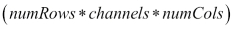. This would mean that the outer loop runs only once and we call the `Mat::ptr()` method once to fetch all the pixels of the image in a single call. And if our matrix hasn't been flattened, then `image.ptr<uchar>(i)` will be called for each row that makes it a total of `numRowstimes`. This is also the reason that flattening a matrix is more efficient in terms of time taken.

Let's put together the code for the flattening and traversal of the image to get a complete picture of using the `pointer` method for Mat object traversal:

```void scanImage(Mat& image) {
int channels = image.channels();
int num_rows = image.rows;
int num_cols = (image.cols * channels);

if (image.isContinuous()) {
num_cols *= num_rows;
num_rows = 1;
}

for (int i = 0; i < num_rows; ++i) {
uchar* row_ptr = image.ptr<uchar>(i);
for (int j = 0; j < num_cols; ++j) {
// Perform operations on pixel value row_ptr[j]
}
}
}
```

So, in summary, the `Mat::ptr()` method essentially works by fetching the data one row at a time. In that sense, the access method here is sequential: when the data of one of the rows is fetched, we can go over the contents of only that particular row. Accessing a new row necessitates a new fetch call. Flattening the data matrix is just a way to speed up computation, which works by bringing in all the data in a single fetch. This might not be the most aesthetic way of doing things. Your code may sometimes be difficult to understand and/or debug, especially when it comes to handling multi-channel images (you need to know exactly how many columns to skip per pixel while traversing a row). Now, this is where our second approach comes in.

This method relies on the `Mat::at()` method. As per the OpenCV documentation, the `at()` method returns a reference to any specified array element. The pixel whose value we are interested in is specified via the row and column index. This approach provides us with a random access to the data matrix. Let's look at an example code in action that uses the `at()` method to access pixel values. In the following code snippet, assume that `I` is a single-channel, grayscale image:

```for( int i = 0; i < I.rows; ++i) {
for( int j = 0; j < I.cols; ++j) {
// Matrix elements can be accessed via : I.at<uchar>(i,j)
}
}
```

The code looks much simpler, more compact, and easier to read than the earlier approach. We have a couple of `for` loops: the outer loop (with index variable `i`) which iterates over the rows and the inner loop (with index variable `j`) that goes over the columns. As we move over each pixel, we can access its value by calling `I.at<uchar>(i,j)`.

But what about the case when our image is multi-channeled? Let's say that we have a three-channel RGB image that we need to traverse. The code would have a very similar structure but with minor differences. Since our image is now three-channeled, the  `uchar` data type will not be appropriate for the pixel values. The solution is presented in the following code snippet:

```for( int i = 0; i < I.rows; ++i) {
for( int j = 0; j < I.cols; ++j) {
/**
* The B, G and R components for the (i, j)-th pixel can be accessed by:
* I.at<Vec3b>(i, j)
* I.at<Vec3b>(i, j)
* I.at<Vec3b>(i, j)
**/
}
}
```

The first thing you notice about the code is the use of what seems like a new OpenCV type named `Vec_3b`. All you need to know about `Vec_3b` at this point is that it stands for a vector of three byte values, that is, a vector of three numbers between 0 and 255 (inclusive). And that seems to be the perfect data type for representing what a pixel stands for in a three-channel RGB image (OpenCV always has the right tools made available to its users!). Now that we have established that the type of each value in the data matrix is `Vec_3b`, which means that the `at()` method returns a reference to `Vec_3b`, we can access the individual elements within `Vec_3b` using the `[]` operator, just like a C++ array or vector. Now, recall that when we discussed about image channels, we said that the OpenCV stores the R, G, and B components in the reverse order. This would mean that the zeroth, first and second elements of `Vec_3b` would each refer to the blue, green, and red components of the pixel, respectively. You should be extra careful about this fact as it can be a potential source of errors in your code.

Now, the library has gone a step further to provide another level of convenience for its users. Using the previously mentioned approach, we have to write the name of the data type `Vec_3b` every time we want to access the value for a particular channel of a particular pixel. In order to avoid that, OpenCV provides us with a template class named `Mat_`. As always, we demonstrate its use via an example code snippet:

```Mat_<Vec3b> _I = I;
for( int i = 0; i < I.rows; ++i) {
for( int j = 0; j < I.cols; ++j ) {
/**
* The B, G and R components for the (i, j)-th pixel can be accessed by:
* _I(i, j)
* _I(i, j)
* _I(i, j)
**/
}
}
```

The first thing we do is declare an object of the `Mat_` class and initialize it with our original Mat object. `Mat_` is a thin template wrapper over the `Mat` class. It doesn't contain any extra data fields in addition to what is available with the Mat object. In fact, references to the two classes (`Mat` and `Mat_`) can be converted to each other. The only advantage `Mat_` offers is the notational convenience of having to skip writing the data type every time we have to access a pixel (this is because the data type has been specified during declaration of the `Mat_` object itself).

As stated earlier, the `Mat::at()` method is suited for random access (it requires both the row and column index), the code is much more readable and clean, but it is slower than the pointer-based approach because the `at()` method does some range checks each time it is called.

We combine both the code snippets for single as well as multi-channel traversal using `Mat::at()` and encapsulate that within a single C++ function:

```void scanImage(Mat& image) {
int channels = image.channels();

if (channels == 1) {
for( int i = 0; i < I.rows; ++i) {
for( int j = 0; j < I.cols; ++j) {
// Matrix elements can be accessed via : I.at<uchar>(i,j)
}
}
}
else if (channels == 3)  {
for( int i = 0; i < I.rows; ++i) {
for( int j = 0; j < I.cols; ++j) {
// The B, G and R components for the (i, j)-th pixel can be
// accessed by:
//   I.at<Vec3b>(i, j)
//   I.at<Vec3b>(i, j)
//   I.at<Vec3b>(i, j)
}
}
}
}
```

This concludes our section on image traversals. But before we move on to the next topic, a few final words on Mat object traversals. We have gone over a lot of different methods to achieve what seems like a very basic task. We have seen the sequential-pointer approach and the random-access technique using the `Mat::at()` method. Personally, I tend to lean towards the latter due to its aesthetic appeal and a clear distinction between single and multichannel images that leaves no room for confusion. It's usually also safer, due to the range checks that we've mentioned before, and it's also easier to access the surrounding pixels if you need them for processing (something that we would be doing quite a lot from Chapter 2, Image Filtering.

Most of the example programs in the remainder of this book will stick to this too. However, you are encouraged to try out the former approach too, if and when you feel like, and compare the results with the ones shown in the text.

Now, we have been traversing Mat objects and images for quite some time now but haven't really been doing any sort of tangible processing with them. You will have noticed that when it came to the section of code where we had the chance to actually access and/or modify the pixel values, we stopped and hid behind those boring comment blocks that did nothing but tell us more theory about how to code. Very soon, in the next few sections, we are going to remove those comments and fill up that space with some actual code that performs some simple, yet cool transformations on our images!

## Image enhancement

This section is all about performing some form of computation or processing on each pixel. Since this is the beginning of the book and we are dealing with the basics, we'll let the computations be fairly simplistic for now. The more complex algorithms will be saved for the next chapter. In addition to being simplistic in nature, the computations will also involve all the pixels undergoing the same nature of transformations. The transformation function to be applied to every pixel is dependent only on the value of the current pixel. Putting it mathematically, such transformation functions can be represented as follows:

s=T(r)

Here, s is the output pixel value and r is the input. The transformation function, T, also known as the gray-level or intensity transformation function, can be thought of as a mapping between the input and output pixel values. Essentially, the pixel value at the `(i, j)` position in the output image is dependent only on the pixel value at the same `(i, j)` position in the input image. Hence, you do not see any dependency of coordinate positions `(i, j)` in the transformation function, just the pixel values `s` and `r`. However, these transformations are pretty naive to assume such a simple pixel-dependency model. Most of the image processing techniques work with a neighborhood of pixels around the `(i, j)` pixel. It is due to this reason that grayscale transformations are simple. However, they are a good starting point for our journey on image processing.

Assume that we are dealing with a grayscale image (even in the case of a color image, the R, G, and B channels can be treated separately and independently as three grayscale images). T is applied to each and every pixel in the input image to yield the output. By changing the nature of T, we can get different forms of transformations. The names of some of the transformations that we'll discuss and ultimately implement have been listed as follows:

• Linear transformations­:

• Identity

• Negative

• Logarithmic transformations:

• Log

• Inverse log or exponential

At this point, you can probably see the path laid out in front of you. We implement these grayscale transformations by traversing the data matrix by taking help from the arsenal of techniques we have developed in the previous section, and we apply the transformation function independently at each pixel to get the resultant image. While this approach is perfectly correct, there is yet a scope for optimization.

## Lookup tables

Consider an image 1000 pixels high and 800 pixels wide. If we are to follow the aforementioned approach of visiting each pixel and performing the transformation T, we will have to perform the computation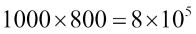times. This number increases in direct proportion to the size of the image.

At the same time, we also know that the value of each pixel only lies between 0 and 255 (inclusive). What if we can pre-compute and store the transformed values s=T(r) for values s=T(r) for r∈{0,1,2,...,255}, that is, for all possible values of the input. If we do so, then irrespective of the dimensions (number of pixels) in our input image, we will never need more than 256 computations. So, using this strategy, we traverse our matrix and do a simple lookup of the pre-computed values. This is called a lookup table approach (often abbreviated as LUT). Using LUT affords us with yet another benefit with regards to implementing our transformations. The logic/code for image traversals is independent of the logic for the actual computation of the grayscale transformation. This decoupling makes our code more readable, easy to maintain, and scale (add more and more transformations to our suite). Let's have a look at an example to elucidate what I'm trying to convey:

```vector<uchar> getLUT() {
/**
* This function holds the implementation details of a specific
* grayscale transformation
*/
}

void processImage(Mat& I) {
vector<uchar> LUT = getLUT();
for (int i = 0; i < I.rows; ++i) {
for (int j = 0; j < I.cols; ++j)
I.at<uchar>(i, j) = LUT[I.at<uchar>(i, j)];
}
}
```

As you can see, we have used a combination of `LUT` and the random-access using `Mat::ptr()` for matrix traversal to define a framework for implementing grayscale transformations. The `getLUT()` method basically returns the lookup table as a C++ vector. Usually, the vector is constructed in such a way that the input value `r` can be used as an index into the `LUT` and the value stored as the vector element is the target value `s`. This means that if I want to know what value the input intensity `185` is mapped to, we would simply call `LUT` to get it. Naturally, `LUT` will have a size of `256` (so that the indices range from `0` to `255`, thereby covering all possible input values). Now, while we traverse the data matrix in the `processImage()` method, we take the intensity value of each input pixel, query the `LUT` vector to know the desired output pixel value, and assign the new value. If you remember the section where we talked about the internals of Mat, we mentioned that Mat objects are always passed by reference and then called, and the caller function, are working with the same underlying data matrix. So, in the implementation framework that we have presented here, the same matrix will be modified and overwritten. If you want to have the original image preserved, you should create a new matrix by cloning and passing the cloned copy to the `processImage()` function. I guess you might have begun to appreciate the importance of learning about the internal workings of Mat now!

Let's take a moment to pause and think about what we've accomplished so far and the path that lies ahead. We have learnt about traversal of data matrix of Mat objects using a couple of different approaches. Then, to demonstrate the utility of such traversals, we introduced the concept of grayscale transformations, and talked about the design of a framework that would allow us to implement such transformation techniques.

Going forward, in the next section, when we discuss these transformations in detail, you will realize that each one of them modifies the image in its own characteristic way. They are meant to act upon certain aspects of the image and bring out the details that they are designed to exploit. That is the reason that these transformations are also referred to as image enhancement techniques. Very soon, we are going to demonstrate the different kinds of enhancements that you can bring about in your images by just transforming pixel values in accordance to a predefined function. Everyone, I guess at some point in time, has used a web or mobile-based photo/video editing application. You might recall that there is a dedicated section in such applications whose purpose is to apply these enhancements to images. In common Internet terminology, these are often referred to as filters (for example, Instagram filters). As we take you through these grayscale transformations, you will realize that at the most basic level, this is what the fancy filters really are. Of course, to design a full-scale, production-level image filter would involve a lot of different steps other than the basic s=T(r), but grayscale transformations do act as a good starting point. Without any further ado, let's learn about these transformations while building our own simple (yet cool) set of image filters by the side.

## Linear transformations

As mentioned previously, we will be discussing two broad categories of grayscale transformations: linear and logarithmic. We will start with linear transformations first.

When it comes to grayscale transformations, there are broadly two types of transformations that are widely discussed:

• Identity

• Negative transformation

In theory, you can make up as many arbitrary linear transformations that you want, but for the purpose of this book, we will restrict ourselves to just the two.

### Identity transformation

The identity transformation maps each input pixel to itself in the output. In other words:

T(r)=r

Obviously, this does nothing exciting. In fact, this transformation doesn't do anything at all! The output image is the same as the input image, because every pixel gets mapped to itself in the transformation. Nevertheless, we discuss it here for the sake of completeness.

Implementing a lookup table for identity transformations shouldn't be a hassle at all:

```vector getIdentityLUT() {
vector<uchar> LUT(256, 0);
for (int i = 0; i < 256; ++i)
LUT[i] = (uchar)i;
return LUT;
}
```

The first line of the function declares and initializes the C++ vector that is going to serve as our lookup table. This same vector is then computed and returned by the function. We had discussed earlier (while talking about lookup tables) that the size of `LUT` will, in practically all cases, be 256 for the 256 different intensity values in a grayscale image. The `for` loop traverses over `LUT` and encodes the transformation. Notice that `LUT[i]=i` will map every input pixel to itself, thereby implementing the identity transformation.

As stated earlier, one of the secondary benefits of using a lookup table is that it modularizes your code and makes it cleaner. The preceding snippet that we showed for identity transformations only computes and returns the lookup table. You can use a matrix traversal method after this to actually apply the transformation to all the pixels of an image. In fact, we demonstrated this framework in our section on Lookup Tables.

```void processImage(Mat& I) {
vector<uchar> LUT = getLUT();
for (int i = 0; i < I.rows; ++i) {
for (int j = 0; j < I.cols; ++j)
I.at<uchar>(i, j) = LUT[I.at<uchar>(i, j)];
}
}

void main() {
Mat processed_image = image.clone();
processImage(processed_image);

imshow("Input image", image);
imshow("Processed Image", processed_image);
waitKey(0);

return 0;
}
```

Note that while invoking the `processImage()` method to do our bidding, we have passed it a clone of the matrix that we just read from the input. This is just so that we are able to compare the changes that the processing has made on our input (not that there is any, in this particular case!). From the next transformation onwards, we are not going to write down the full detailed code (along with the `processImage()` and `main()`). We'll focus on the computation of the lookup table because that is what varies from one transformation to the next.

### Negative transformation

The negative transformation subtracts `255` from the input pixel intensity value and produces that as an output. Mathematically speaking, the negative transformation can be expressed as follows:

s=T(r)=(255-r)

This means that a value of `0` in the input (black) gets mapped to `255` (white) and vice versa. Similarly, lighter shades of gray will yield the corresponding darker shades from the other end of the grayscale spectrum. If the range of the input values lie between `0` and `255`, then the output will also lie within the same range. If you aren't convinced, here is some mathematical proof for you: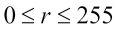(Assuming the input pixels are from an 8-bit grayscale color space)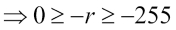(Multiplying by-1)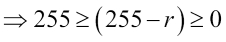(Adding 255)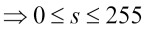Some books prefer to express the negative transformation as follows:

s=T(r)=(N-1-r)

Here, N is the number of grayscale levels in the color space we are dealing with. We have decided to stick with the former definition because all the color spaces we'll be dealing with in this book will involve values between 0 and 255. Hence, we can avoid the unnecessary fastidiousness.

The implementation of a lookup table for the negative transform is also fairly straightforward. We just replace the `i` with `(255-i)`:

```vector<uchar> getNegativeLUT() {
vector<uchar> LUT(256, 0);
for (int i = 0; i < 256; ++i)
LUT[i] = (uchar)(255 - i);
return LUT;
}
```

Let's run the code for negative transformation on some images and check what kind of an effect it produces: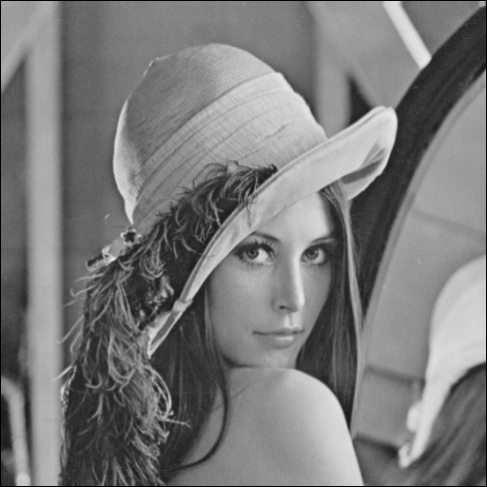The preceding image serves as our input image and the output (negative transformed image) is as follows:.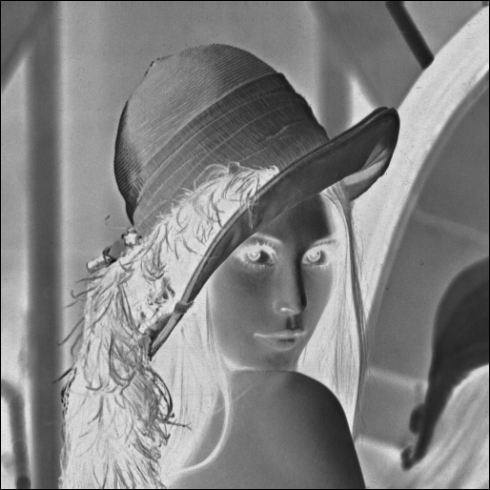You can notice the darker pixels that make up the woman's hair (and feathers on the cap) have been transformed to white, and so have the eyes. The shoulders, which were on the fairer side of the spectrum, have been turned darker by the transform. You can now start to appreciate the kind of visual changes that this conceptually simple transformation seems to bring about in images! We iterate, once again, that the image manipulation apps, at the most basic, operate on similar principles.

If you are wondering who the lady in the image is, she goes by the name, Lena. Rather surprisingly, Lena's photograph with her iconic pose has become the de facto standard in the image processing community. A lot of literature in the field of image processing and computer vision uses this photo as an example to demonstrate the workings of some algorithm or technique. So, this is not the first time you'll be seeing her in this book!

Before we finish the section on linear transformations, there is one more thought that I would like to leave you with. You might find some texts out there that like to visualize these transformations graphically as a plot between the input and output pixels. The reason that such visualization is made is because not all linear transformations are as simple as the ones discussed here. We will briefly discuss piecewise linear transformations, where a graphical plot provides a convenient medium to analyze the transformation. But, before that, you will find such a plot for both the identity and the negative transformation drawn on the same graph: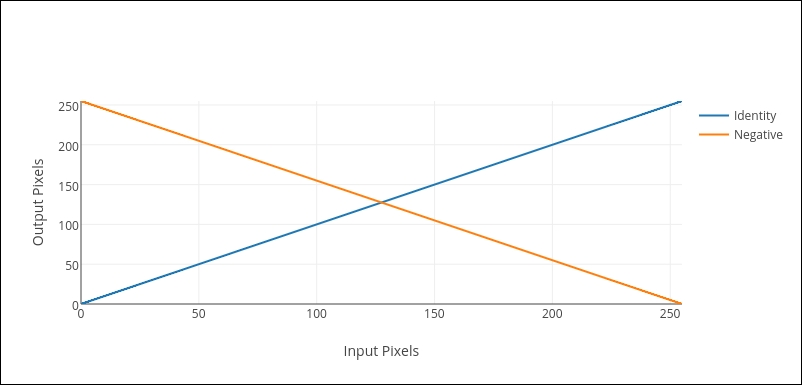Both the linear transformations that we have discussed so far--identity and negative--are fairly trivial ones. You can have much more complicated forms for `s=T(r)`. For example, instead of the transformation function being linear throughout the entire domain (0 to 255), we can make it piecewise linear. That would mean splitting the input domain into multiple, contiguous ranges and defining a linear transformation for each range, something along the lines of this: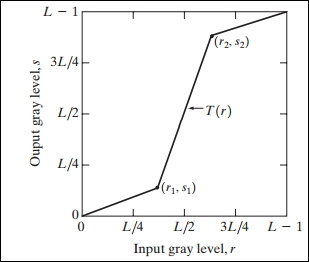If you are wondering what purpose such a transformation achieves, the answer to that is contrast enhancement. When we say that an image has poor contrast, we mean that some (or all) parts of the image are not clearly distinguishable from their surroundings and neighbors. This happens when the pixels that make up the part of the image all belong to a very narrow band of intensity values. In the graph, consider the portion of the input intensity values that lie around L/2. You will notice that the narrow range of input pixels are mapped to a much wider range of output intensity values. This has been made possible by the steep line (greater slope), which defines the linear transformation around that region. As a result, all pixels that have intensity values within that range will get mapped to the wider output range, thereby improving the contrast of the image.

Now, astute readers might have realized that the shape of the piecewise linear transformation is dependent on the position of the points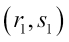and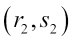So, how do we decide the location of the two points? Unfortunately, there is no single, specific answer to this question. As you will learn throughout the course of this book, in most cases, there can be no global correct answer for the selection of such parameters in image processing or computer vision. It depends on the kind of data (within the domain of computer vision, data often equates to images) that we are given to work with.

It would be a good exercise to try and implement the lookup table for a piecewise linear transformation. You can control the shape of the curve by varying the position of the two points and try to see what kind of an effect it has on the algorithm performance.

## Logarithmic transformations

Having discussed linear transformations in the last section, we step into logarithmic transforms now. You will notice that they are mathematically more involved than their linear counterparts. Again, we'll be discussing two different types of enhancement techniques under logarithmic transforms:

• The log transform

• The exponential (or inverse log) transformation

### Log transformation

Simply put, the log transform takes the (scaled) logarithm of every input pixel intensity value. Let's put it down in terms of a mathematical equation: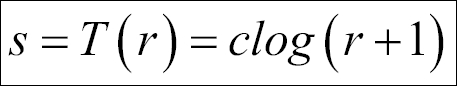First, note that the input intensity values have all been incremented by 1 (r+1). This is because our input values vary from 0 to 255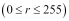and the logarithm of 0 is not defined. Secondly, there has been no mention regarding the base of the logarithm. Although conceptually the value of the base doesn't really matter (as long as it's kept same throughout the computation), for all practical purposes, we will assume it to be 10. So, when we write log, we actually mean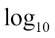. Thirdly, you must be wondering about the constant c in the formula. What's it doing there? To answer that question, we need to know the range of output values for log(r+1) when. To help us, I have plotted a graph of the function log(r+1):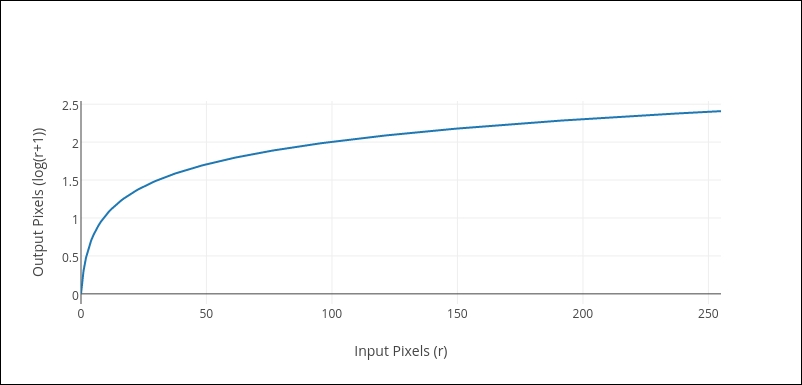As r varies from 0 to 255, log(r+1) ranges from 0 to 2.4. It's in the nature of a logarithmic function to compress the range of input data, as is evident here: an input range spanning 256 values has been compressed to a mere range of 2.4. Does this mean that the output image will have a grayscale range of merely two or three values? It had better not, otherwise, the only thing you'll be able to see is complete darkness! This is where the multiplicative constant c comes into the picture. The role of the multiplier is to make the log-transformed pixel values span the entire range of 256 grayscale levels available for the output image. The way it's done is by choosing a value of c so that the maximum intensity available in the input image gets mapped to 255 in the output. This means that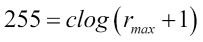, further implies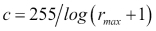. Often, for sufficiently large and contrast-rich images, it so happens that the maximum intensity in the input image is 255, that is,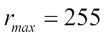. In such cases, the value of the multiplier is c=105.886.

So far, we have been treating the log transformation in a highly mathematical context. Let's see what happens if we actually apply it to images. The image is made up of two horizontal bands. The first band depicts the grayscale color space from 0 (black) on the left and all the way up to 255 (white) on the right end of the spectrum:The next band depicts the log transform of the corresponding grayscale values (again, from 0 to 255, as we move from left to right). A comparison between the two should give you an idea of what the log transform does to the grayscale spectrum. A glance will tell you that the log-transformed band is much more brighter than its counterpart. Why does this happen?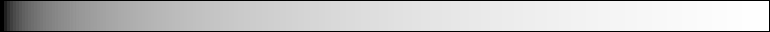To give you a better perspective, intensity values of 0 and 15 in the input are mapped to 0 and 127 in the output. This means that if there are two adjacent pixels with intensities 0 and 15 in the input image, both of them would be almost indistinguishable. Human eyes will not be able to perceive such a subtle change in the grayscale intensity. However, in the log-transformed image, the pixel with the intensity value of 15 gets converted to 127 (which lies in the middle of the grayscale spectrum). This would render it clearly distinguishable from its neighbor, which is still completely black!

The exact opposite phenomenon takes place at the other end of the spectrum. For example, pixels with intensities of 205 and 255 are mapped to 245 and 255 by the log transform. This means that a significant difference of 50 in the grayscale spectrum has been reduced to a mere gap of 10. So, the log transform essentially magnifies the differences in intensity of pixels in the lower (darker) end of the grayscale spectrum at the cost of diminishing differences at the higher (brighter) end (notice the steepness of the log curve in the beginning and how it flattens as it reaches the end). In other words, the log transform will magnify details (by enhancing contrast) in the darker ends of the spectrum at the cost of decreasing the information content held by the higher end of the spectrum.

Now that you have an idea of the kind of changes brought forth in grayscale by a log transform, it's time we take a look at some real examples. If you have ever used a camera, you would know that pictures, when taken against a source of light (such as the sun or an artificial source such as a bulb or a tube-light) appear darker. The following image is an example of an image taken under such lighting conditions: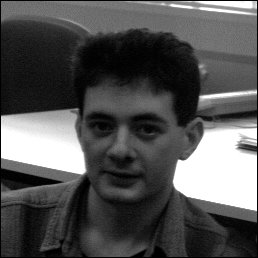Now try to think of what would happen if we applied the log transform to such an image. We know that a log transform would enhance details from the darker region at the cost of information from the brighter regions of the image. The log-transformed image is shown next. We can see that the darker regions in our original image such as the face and the back of the chair in the background have been rendered more rich in contrast. On the other hand, there has been a significant loss in detail from the brighter segments, such as the table behind the person. This proves that the log transform can be effective in editing pictures that have been captured against the light source by digging out contrast information from the darker regions of an image at the cost of the brighter segments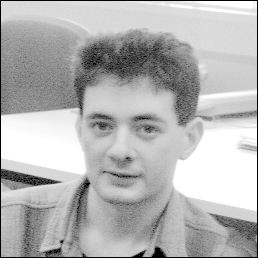Before we move on to the implementation, let's see one more application where a log transform may be considered useful. There are some scientific disciplines where we might come across patterns such as the one depicted in the following image: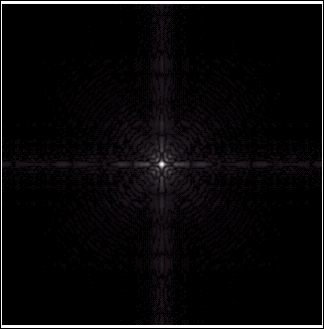This image represents a pattern made by a light source on a dark background. More specifically, this is the representation of the Fourier transform of an image. As you can see, there definitely seems to be a pattern, but it's not clearly visible in its native form. We need a way to magnify and enhance these variations that are too subtle to be detected by the naked eye. Log transform to the rescue once more!

The log-transformed image is shown adjacent to the original one. We can observe the pattern quite clearly here: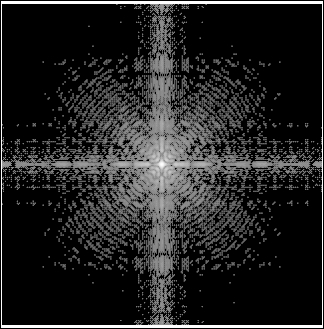Now that we have familiarized ourselves with the mathematics behind the log transform and seen it operate on and transform images, we come to the most exciting part where we attempt to mimic their behavior via our OpenCV/C++ code. In accordance with the protocol we have adhered to so far, we first show the code that generates a lookup table for the log transform:

```#include <cmath>

vector<uchar> getLogLUT(uchar maxValue) {
double C = 255 / log10(1 + maxValue);

vector<uchar> LUT(256, 0);
for (int i = 0; i < 256; ++i)
LUT[i] = (int) round(C * log10(1+i));
return LUT;
}
```

We notice that the lookup table function is a bit different and slightly more involved than the ones we have discussed thus far. This is mainly because it requires a parameter to operate upon the maximum pixel intensity value in the input image. Recall the description of the log transform, where we discussed that the value of the multiplicative constant c is calculated on the basis of the maximum intensity value,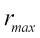, among the input pixels--. Knowing this fact, the remainder of the function is similar in structure to what we have seen so far.

Now, since the function that returns our lookup table (`getLogLUT()`) requires an additional parameter, we would have to make appropriate changes to the code that makes calls to it, that is, our `processImage()` method. The code for our `processImage()` method is as follows:

```void processImage(Mat& I) {
double maxVal;
minMaxLoc(inputImage, NULL, &maxVal);
vector<uchar> LUT = getLUT((uchar) maxVal);

for (int i = 0; i < I.rows; ++i) {
for (int j = 0; j < I.cols; ++j)
I.at<uchar>(i, j) = LUT[I.at<uchar>(i, j)];
}
}
```

The one thing that is noteworthy in the preceding snippet is the use of a method named `minMaxLoc()`. As per the documentation, the function is used to find the minimum and maximum element values and their positions within the array (and by array here, we are referring to a Mat object). The first argument is, of course, the name of the Mat object. The second and the third arguments are the pointers to the minimum and maximum elements, as computed by the function. We have passed the second argument as `null` because we aren't really interested in the minimum value for now. Apart from the call to `minMaxLoc()`, the structure of the remainder of `processImage()` should be familiar to you.

The implementation technique that we have employed for implementing the log transform has followed the framework that we established early on: lookup tables and image traversals. However, as we progress through this book, you will come to appreciate the fact that often, there are multiple ways to reach the same endpoint while implementing your programs in OpenCV. Although this is true for programming in general, we want to focus on how OpenCV provides us with options that allow us to perform and (more often than not) simplify tasks that otherwise would take a lot of tedious steps (iterations) to achieve. To that end, we will present another technique using OpenCV to compute the log transformation for images.

Like always, we first begin by including the relevant headers and namespaces:

```#include <opencv2/core/core.hpp>
#include <opencv2/highgui/highgui.hpp>
#include <opencv2/imgproc/imgproc.hpp>

using namespace std;
using namespace cv;
```

Barring the declarations, our code that initially spanned a couple of user-defined functions and a `main()` class has now been essentially reduced to five lines of code that do all the work! Nowhere do we explicitly traverse any data matrix to modify pixel values based on some predefined transformation functions. The native methods that we use do that in the background for us. Have a look at the following code:

```int main() {
Mat processed_image;

input_image.convertTo(processed_image, CV_32F);
processed_image = processed_image + 1;
log(processed_image, processed_image);
normalize(processed_image, processed_image, 0, 255, NORM_MINIMAX);
convertScaleAbs(processed_image, processed_image);

imshow("Input image", image);
imshow("Processed Image", processed_image);
waitKey(0);

return 0;
}
```

The five major functions used have been described in detail as follows:

1. The `convertTo()` function converts all the pixel values in the source array (Mat object) into the target data type. The destination array (which will store the corresponding converted pixel values) is the first and the target data type is the second argument that is passed to the function. Since we will be dealing with logarithmic calculations, it is best to shift to `float` as our data type.

2. The next statement after the `convertTo()` call increments all the pixel values by one. Recall that before applying the log operator, all pixel values have to be incremented by one as per the formula s=T(r)=clog(r+1). This is to avoid possible errors when a 0 is passed to a `log` function. The key thing to notice here is how operator overloading elegantly allows us to operate on entire data matrices with a single algebraic command.

3. The `log()` function calculates the natural logarithm of all the pixel values. After this step, what we have calculated so far would be `log(r+1)` for all pixels.

4. The `normalize()` method performs the same function as done by the multiplicative constant `c` in the formula T(r)=clog(r+1). That is, it makes sure that the output lies in the range of 0 to 255 (as specified in the arguments passed to it). The way it does that is by applying the MIN-MAX normalization (again, another argument passed to it) technique, which is nothing but linearly scaling the data while making sure that the minimum and maximum of the transformed data take certain fixed values (0 and 255, respectively).

5. Finally, we apply `convertScaleAbs()`, which is the antithesis of `convertTo()`: it converts all the pixel values back to 8 bits (`uchar`).

One of the most prominent and striking differences that you will notice with this method is that it completely relies on the functions provided by the OpenCV API. What we have essentially done is avoid reinventing the wheel. Knowing how to traverse data matrices was, no doubt, an important skill to master. However, something as basic as iterating Mat objects becomes tedious, time consuming, and off-topic when we have big and complex computer vision systems to build. In such scenarios, it is good to utilize the features of the library if they have been made available to us. A classic example is the overloading of mathematical operators for the `Mat` class. Imagine if we had to implement a fully-fledged matrix traversal every single time we needed an operation as simple as increment all pixels by 1. To keep things concise and readable in our code and speed up the development cycle at the same time, the library has afforded us the luxury of writing I=I+1, even for the objects of the `Mat` class! Another advantage that we get if we rely on the OpenCV functions as much as possible is that we are guaranteed that the code that runs is heavily optimized and efficient in terms of memory and runtime.

The developers at OpenCV have built as many abstractions over such behind-the-scenes, plumbing operations as is required by programmers like us to seamlessly develop a varied set of applications that falls within the domain of computer vision and machine learning, without having to worry about the intricacies of implementation. This will be a recurrent theme in our book across most of the chapters.

### Exponential or inverse-log transformation

Before we finish this section, we will visit our final transformation that goes by the name of exponential transform. What it does essentially is the complete opposite of the log transform (hence, it is also named inverse-log transform). While the log transform enhanced the pixels in the lower end of the spectrum, the exponential transform does the same for the pixels at the high intensity end of the spectrum. Mathematically, we have the following: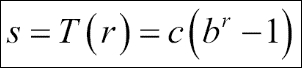Just like computing the log operator essentially involves taking the logarithm of the intensity values of every input pixel, the exponential transform raises a base value b to the power of the input pixel's intensity value. We subtract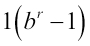so that when the input is 0, the output gets mapped to 0 as well. The constant c plays the same role as in the case of log transform, ensuring that the output lies in the range of 0 to 255. The value of the constant b decides the shape of the transform. Typically, b is chosen to lie close to 1. The following graph depicts a plot of both the log and the exponential transform (b=1.02):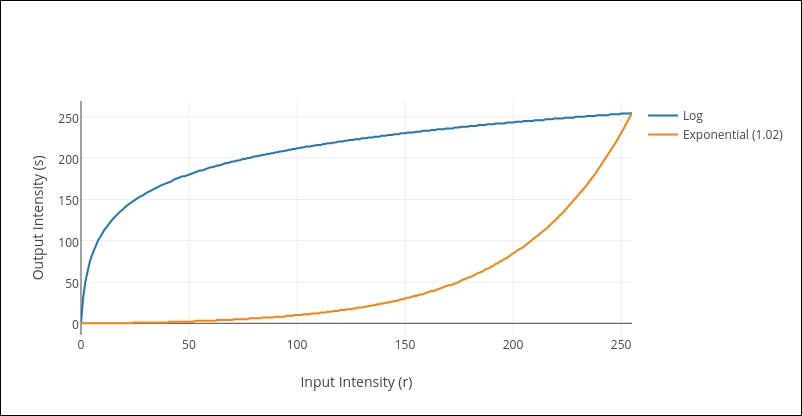The shape of the plots brings out the complementary nature of both the transforms. On one hand, the log transform maps a narrow range of input intensity values at the lower end of the grayscale spectrum to a broader range at the output. On the other hand, the curve of the exponential transform becomes steep at the other end of the spectrum, thereby mapping a narrow range of input values to a much larger range at the output. To further illustrate the dichotomy between the two, the following figure demonstrates the changes that the exponential transform does to a grayscale spectrum. This is similar to the kind of grayscale comparisons that we did for the Log transformation. The following image depicts the original grayscale spectrum from 0 (on the left) all the way to 255 (on the right):You'll find the corresponding spectrum for exponential transform in the following image. Note how the entire spectrum is darker (as opposed to lighter, in the case of log transforms) than the original grayscale band. Using the same line of reasoning that we presented in the section on log transforms, you can deduce why that happens: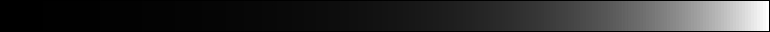As always, we share the code to compute the lookup table for the exponential transform:

```const int BASE = 1.02;
vector<uchar> getExpLUT(uchar maxValue) {
double C = 255.0 / (pow(BASE, maxValue) - 1);

vector<uchar> LUT(256, 0);
for (int i = 0; i < 256; ++i)
LUT[i] = (int) round(C * (pow(BASE, i) - 1));
return LUT;
}
```

The code is exactly similar to the one for calculating log transforms except for the formula. We won't be going over the other functions, such as the traversal and the `main()` function once again. I would strongly suggest you to implement the exponential transform by using the OpenCV functions (and avoid reinventing the wheel by implementing matrix traversals) as we did in the case of log transforms. Take the help of online documentation (OpenCV has excellent online documentation) to find the function that would enable you to take the exponential of pixel values. This is an important skill to learn. There are so many different functions spread across different modules within OpenCV and the documentation is the only reliable and up-to-date source of information. As you go on to develop bigger and more powerful applications, the documentation will be your only ally to help you navigate your way through all the different functions.

Also, we show an example of how the exponential transformation works on images. The following is our original input image:Applying an exponential transform leads us to the following:The overall darkening of the input image is quite apparent!

We have discussed the advantages of using a lookup table-based approach for implementing grayscale transformations. In fact, we have also been implementing all our transformations using a framework based on a combination of computing the lookup table and traversing the data matrix. If this particular combination is so efficient as well as ubiquitous, haven't the OpenCV developers thought of implementing this for us already? If you've followed the trend of this chapter, you would've guessed the answer to the question by now! Yes, OpenCV does have a function that allows you to do exactly that: provide it with a lookup table and a Mat object, and it will transform each pixel of the Mat object on the basis of the rules laid down by the lookup table, and store the result in a new Mat object. What's even better is that the function is named `LUT()`! Let's look at a sample code snippet that uses the `LUT()` method to implement the negative transform.

As we hinted just now, the `LUT()` method requires three parameters:

• The input matrix

• The lookup table

• The output matrix

We have been dealing with the first and the third throughout the chapter. How do we pass the lookup table to the `LUT()` method? Remember that a lookup table is essentially an array (or a vector). We have been treating it as such in all our implementations so far, and we also know that the `Mat` class in OpenCV is more than equipped to handle the processing of one-dimensional arrays. Hence, we would be passing our lookup table as another Mat object. Since our `LUT` is essentially a Mat object, we change our `getLUT()` function as follows:

```Mat getNegativeLUT() {
vector<uchar> lut_array(256, 0);
for (int i = 0; i < 256; ++i)
lut_array[i] = (uchar)(255 - i);

Mat LUT(1, 256, CV_8U);
for (int j = 0; j < 256; ++j)
LUT.at<uchar>(0, j) = lut_array[j];
return LUT;
}
```

Notice that the first three lines are identical to what we have been doing so far-initializing and constructing our lookup table as a C++ vector. Now, we take that vector and transform it into a Mat object having one row and 256 columns and type `CV_8U` (which makes it the perfect container for the elements of a C++ vector of `uchar`). The remainder of the function makes that transition and returns the Mat object as our `LUT`.

Once the `LUT` has been created, applying it is as simple as calling OpenCV's `LUT()` method with all the necessary arguments:

```LUT(input_image, lookup_table, output_image);
```

## Summary

This concludes our first chapter. We have come a long way! We began our discourse on image processing and computer vision by talking about images and how they are represented inside a computing device. We also began our journey into the world of OpenCV by discussing how the library handles image data in its programs, thereby introducing the `Mat` class. A significant portion of the chapter was devoted to learning about how to use the `Mat` class, instantiating objects, learning about its internal structure, and getting intimate with some memory management that takes place under the hood. I hope that, by now, handling images in your code has been demystified for you and you are comfortable dealing with the different forms in which Mat objects appear in the code samples scattered throughout the remainder of the book.

This chapter also served a first taste of some processing that we can perform on images using OpenCV. You learnt a couple of different methods to iterate through the image data stored inside a Mat object, discussing the pros and cons of each. We went on to establish a framework for writing code to help us in the pixel-wise traversal and processing of images. This very framework came to life when we implemented some common grayscale transformations such as negative, log, and exponential transforms. We witnessed what sort of changes these transformations bring forth in our images.

A very important theme that we touched upon briefly in this chapter and would be repeated in the chapters to come is that there are multiple ways to accomplish the same image processing task. We saw that here when we talked about implementing log transformations. One of the alternatives is to implement everything from first principles (reinvent the wheel) and the other is to rely on the functions and APIs provided to us by the OpenCV developers. In the subsequent chapters, we will be relying less on the former and more heavily on the latter. Our approach henceforth will be to explain the theoretical concepts from scratch using the basic principles but demonstrating the implementations using OpenCV functions. We believe that it will give you the best of both worlds.

Finally, as we close off the first chapter, here is what you can expect going forward. We discussed transforms, which were quite simplistic in the way that they operate. Each pixel in the output image was dependent on only a single pixel in the input image. We will discuss some more sophisticated forms of transformations in the next chapter, where the output at a particular pixel location depends not only on the corresponding pixel intensity at the input, but rather on a neighborhood of values. Also, we will learn about a fundamental manner in which such transformations are visualized-using a filter or a kernel. Such a filtering-based approach is extremely common in the image processing and computer vision world and will make a reappearance in more than one chapter! We will also get an opportunity to extend our arsenal of cool image manipulation techniques that we started building in this chapter.